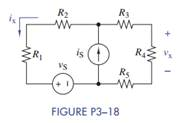### Create an Account

Home / Questions / Formulate mesh-current equations for the circuit in Figure P318 0 b Solve for vx and ix when

# Formulate mesh-current equations for the circuit in Figure P318 0 b Solve for vx and ix when

Formulate mesh-current equations for the circuit in Figure P3−18.

(b) Solve for vx and ix when R= 2:7 kΩ, R2 = 1.5 kΩ, R3 = 680 Ω, R= 2.2 kΩ, R5 = 3:3 kΩ, iS = 10 mA, and vS = 12 V.

(c) Use Multisim to verify your results in part (b) and then find the total power dissipated in the circuit.Jun 15 2020 View more View LessSubscribe To Get Solution